# Mahotas – Element Structure for Dilating Image

In this article we will see how we can set the element structure of the dilate of image in mahotas. Dilation adds pixels to the boundaries of objects in an image, while erosion removes pixels on object boundaries. The number of pixels added or removed from the objects in an image depends on the size and shape of the structuring element used to process the image. In order to dilate the image we use `mahotas.morph.dilate` method.By setting element structure we can increase or decrease the dilating effect on the image.

In this tutorial we will use “luispedro” image, below is the command to load it.

`mahotas.demos.load('luispedro')`

Below is the luispedro image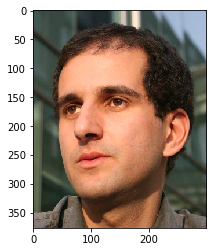Implementation steps :
2. Filter the image
3. Use otsu method for threshold of the image
4. Create a structure of the element with the help of numpy ndarray for binary values
5. Use the element for dilating the image

Below is the implementation

 `# importing required libraries ` `import` `mahotas ` `import` `mahotas.demos ` `from` `pylab ``import` `gray, imshow, show ` `import` `numpy as np ` `  `  `# loading iamge ` `luispedro ``=` `mahotas.demos.load(``'luispedro'``) ` `  `  `# filtering image ` `luispedro ``=` `luispedro.``max``(``2``) ` `  `  `# otsu method ` `T_otsu ``=` `mahotas.otsu(luispedro) ` `   `  `# image values should be greater than otsu value ` `img ``=` `luispedro > T_otsu ` `  `  `print``(``"Image threshold using Otsu Mehtod"``) ` `  `  `# showing image ` `imshow(img) ` `show() ` `  `  `# erode strcture ` `es ``=` `np.array([ ` `        ``[``1``, ``1``, ``1``, ``1``], ` `        ``[``1``, ``1``, ``1``, ``1``], ` `        ``[``1``, ``1``, ``1``, ``1``], ` `        ``[``1``, ``1``, ``1``, ``1``]], ``bool``) ` ` `  `# dilating image ` `dilate_img ``=` `mahotas.morph.dilate(img, es) ` `  `  `# showing dilated image ` `print``(``"Dilated Image"``) ` `imshow(dilate_img) ` `show() `

Output :

`Image threshold using Otsu Mehtod`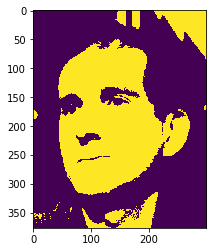`Dilated Image`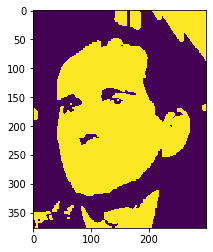Another example

 `# importing required libraries ` `import` `mahotas ` `import` `numpy as np ` `import` `matplotlib.pyplot as plt ` `import` `os ` `  `  `# loading iamge ` `img ``=` `mahotas.imread(``'dog_image.png'``) ` `       `  `# setting filter to the image ` `img ``=` `img[:, :, ``0``] ` ` `  `# otsu method ` `T_otsu ``=` `mahotas.otsu(img) ` `  `  ` `  `# image values should be greater than otsu value ` `img ``=` `img > T_otsu ` ` `  `print``(``"Image threshold using Otsu Mehtod"``) ` ` `  `# showing image ` `imshow(img) ` `show() ` ` `  `# erode strcture ` `es ``=` `np.array([ ` `        ``[``1``, ``1``, ``1``, ``1``], ` `        ``[``1``, ``1``, ``1``, ``1``], ` `        ``[``1``, ``1``, ``1``, ``1``], ` `        ``[``1``, ``1``, ``1``, ``1``]], ``bool``) ` ` `  `# dilating image ` `dilate_img ``=` `mahotas.morph.dilate(img, es) ` `  `  `# showing dilated image ` `print``(``"Dilated Image"``) ` `imshow(dilate_img) ` `show() `

Output :

`Image threshold using Otsu Mehtod`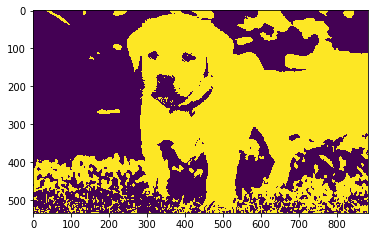`Dilated Image`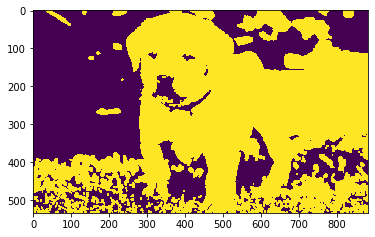Attention geek! Strengthen your foundations with the Python Programming Foundation Course and learn the basics.

To begin with, your interview preparations Enhance your Data Structures concepts with the Python DS Course.

My Personal Notes arrow_drop_upCheck out this Author's contributed articles.

If you like GeeksforGeeks and would like to contribute, you can also write an article using contribute.geeksforgeeks.org or mail your article to contribute@geeksforgeeks.org. See your article appearing on the GeeksforGeeks main page and help other Geeks.

Please Improve this article if you find anything incorrect by clicking on the "Improve Article" button below.

Article Tags :

Be the First to upvote.

Please write to us at contribute@geeksforgeeks.org to report any issue with the above content.